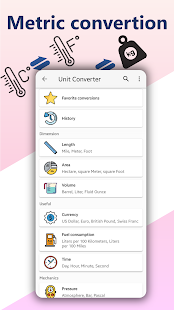PRO Scientific Calculator. This Scientific Calculator contains all the scientific calculations in casio fx 570 es plus and casio 991 es plus and other scientific calculations like casio fx 991 ex plus and casio 570 ex plus, such as trigonometric and hyperbolic calculation, equation solving, system equations solving, anti-trigonometric calculation, integral calculation, power calculation, derivative calculation, logarithmic calculation, nth root calculation, square root calculation, cube root calculation, LCM and GCD calculation, factorial calculation, matrix and vector calculation, π calculation and so on.

Calculator can draw approximately as graphing calculator ti 84, ti 83:
– Cartesian f(x)
– Polar r(t)
– Parametric x(t);y(t)
– Implicit f(x,y)
– Find approximately roots
– Find approximately intersections

PRO Scientific Calculator

This Scientific Calculator contains all the scientific calculations in casio fx 570 es plus and casio 991 es plus and other scientific calculations like casio fx 991 ex plus and casio 570 ex plus, such as trigonometric and hyperbolic calculation, equation solving, system equations solving, anti-trigonometric calculation, integral calculation, power calculation, derivative calculation, logarithmic calculation, nth root calculation, square root calculation, cube root calculation, LCM and GCD calculation, factorial calculation, matrix and vector calculation, π calculation and so on.

Other features
– Basic Calculations
– Mathematical Display: displaying math expression as textbook format
– Equations Solver: the calculator can solve easily any equation
– Complex Number Calculation: output in rectangle and polar form
– Constants
– Numeric Integral & Derivative Calculation
– Fraction and mixed fraction calculation
– Unit converter
– Math formulas
– Calculator ti 83, graphing ti 84, ti 89 calculator

What’s New
– Multi graph workspaces
– Math camera (Need CAMERA permission)
– Removed automatically duplicate history.
– Improved sum function.
– Fixed some bugs

Screenshots

••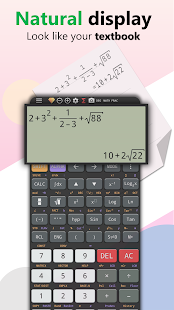•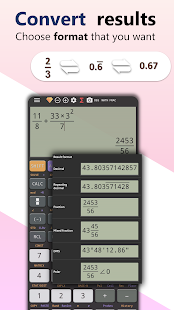••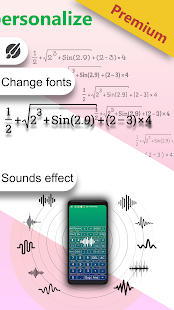•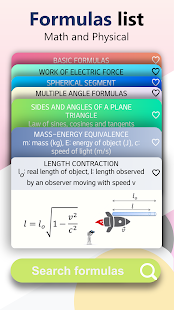•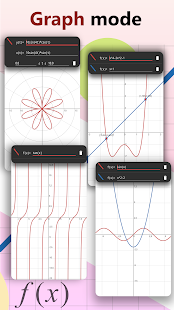•